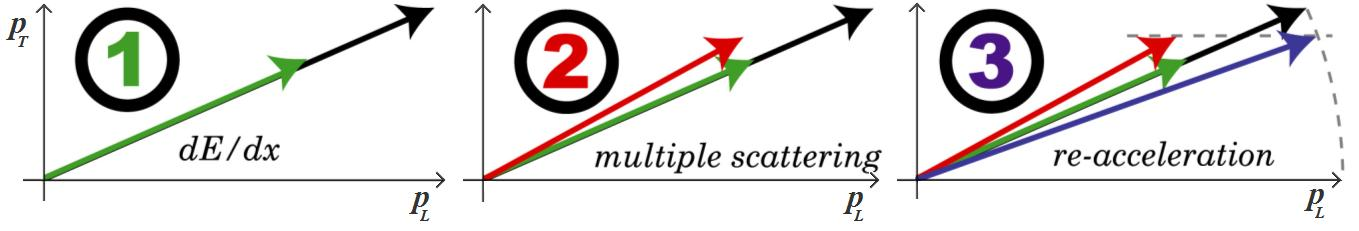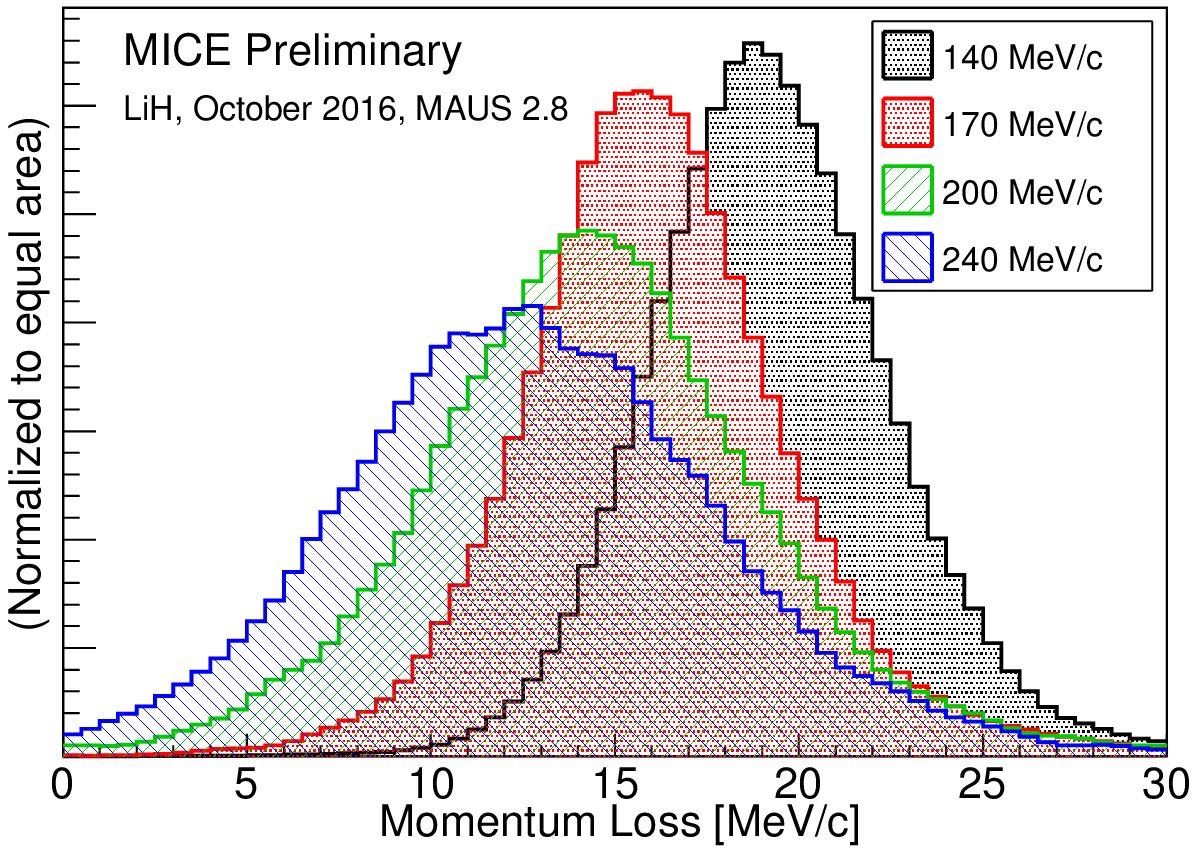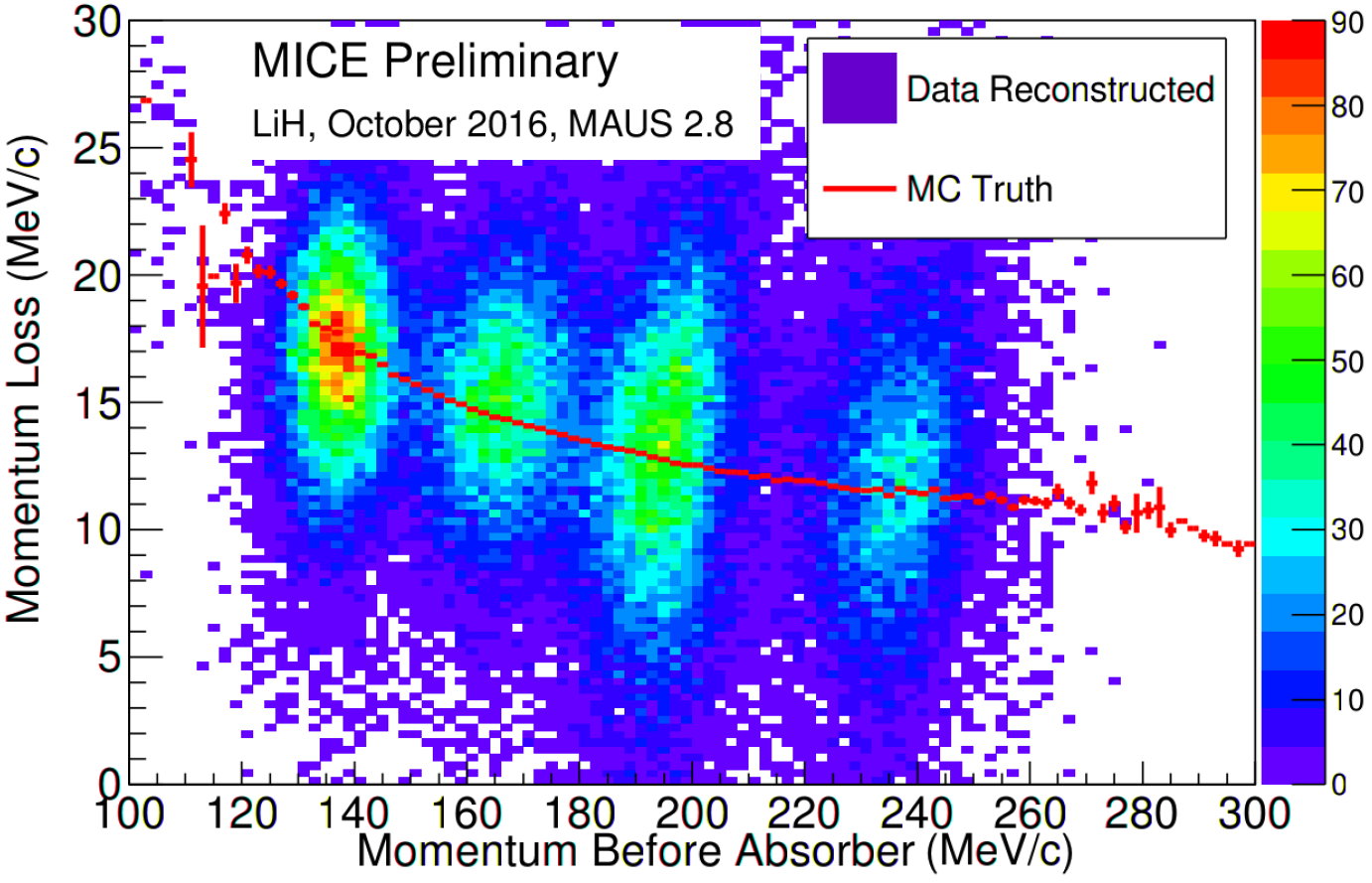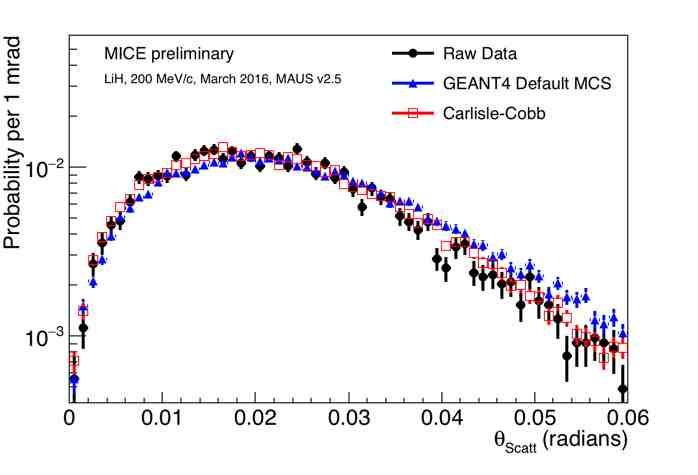## MICE - SCATTERING 04 Apr 2017 Yes -

Muons scatter from the nuclei in the scattering medium. In general, the energy loss occurs from both transverse and parallel components of the momentum (relative to the beam axis). In the future, re-acceleration along the beam axis using RF cavities will cause the focusing of the muon beam. This is ionisation-cooling and re-acceleration.The black vector is the initial momentum of the incoming muon. The green vector represents the average reduced particle momentum due to energy loss in the absorber. The red vector shows the maximum angular deviation in the 'wrong' way for focusing. With RF acceleration along the beam axis, there will be an overall focusing effect (from the black vector to the blue vector).

The angle of deviation, θ, of a trajectory in a scattering process can be described kinematically by the equation

cos (θ) = ab.(M/m) – c.(M/m)2

where a, b, and c are functions of the velocities of the incoming muon of mass m, the scattered muon, and the recoiling nucleus, mass M. Better focusing of the muon beam occurs if the angles of deviation are minimised, so the scattering medium should consist of light nuclei. Data on muon trajectories have been collected with Lithium Hydride, and liquid hydrogen will be used in the near future.

This next figure shows the loss in momentum of individual muons due to scattering processes in Lithium Hydride, binned into 1-d histograms. The initial nominal momentum of the muon beam is shown in the insert top right.This next figure is an intensity plot that bins the loss in momentum of each muon due to scattering processes in Lithium Hydride as a function of its initial momentum in a 2-d histogram. The intense patches occur around the nominal momenta of the incoming muon beams. The red curve indicates the expected average momentum loss.This next figure shows the distribution of scattering angles of 200 MeV/c muons due to the muon beam traversing the lithium hydride absorber. This is compared with: the convolution between the data obtained without the absorber and the GEANT4 prediction of scattering in lithium hydride; and the convolution between the data obtained without the absorber and the Cobb-Carlisle prediction of scattering in lithium hydride.Forward to next item

Contact: Tucker, Mark (STFC,RAL,PPD)